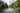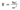## The Hagen Poiseuille Equation

The Hagen-Poiseuille equation describes the relationship between pressure, fluidic resistance, and flow rate. This equation serves as the fundamental tool for fluidics engineers to predict how a Flow Circuit will behave under certain conditions. For instance, if the engineer plans to run a device at a fixed flow rate with a specific fluid with a known viscosity, he can calculate the pressure response at any point in the flow path. This pressure calculation could be critical to ensure a device interface stays below a given design pressure. Backpressure also impacts the amount of fluid creep down unintended channels, so it is important to understand and characterize throughout the entirety of a cartridge run. The Hagen-Poiseuille equation states that pressure is equal to the flow rate multiplied by the fluidic resistance:Fluidic resistance is proportional to the fluid viscosity, the cross-section of the channel, and the amount of fluid currently in that channel. This implies that as fluid fills a channel at a fixed flow rate, the pressure will rise continuously. Conversely, if pumping at constant pressure, the flow rate will decrease continuously. The equation for resistance depends on the cross-section geometry. For a full round pipe the equation is given as:Where µ is dynamic viscosity, L is the length of the filled channel, and r is the pipe radius.

Because a Flow Circuit changes state several times throughout a run with multiple fluid viscosities or varying levels of fill, it is impossible to solve a circuit with a single equation. Rather, the equation needs to be applied at every moment throughout a run to adequately model the flow response. Flow Circuits is the only platform in the world that provides the tools to model your circuit, define its protocol, and simulate its performance from start to finish. Get Started with solving your Flow Circuits today.